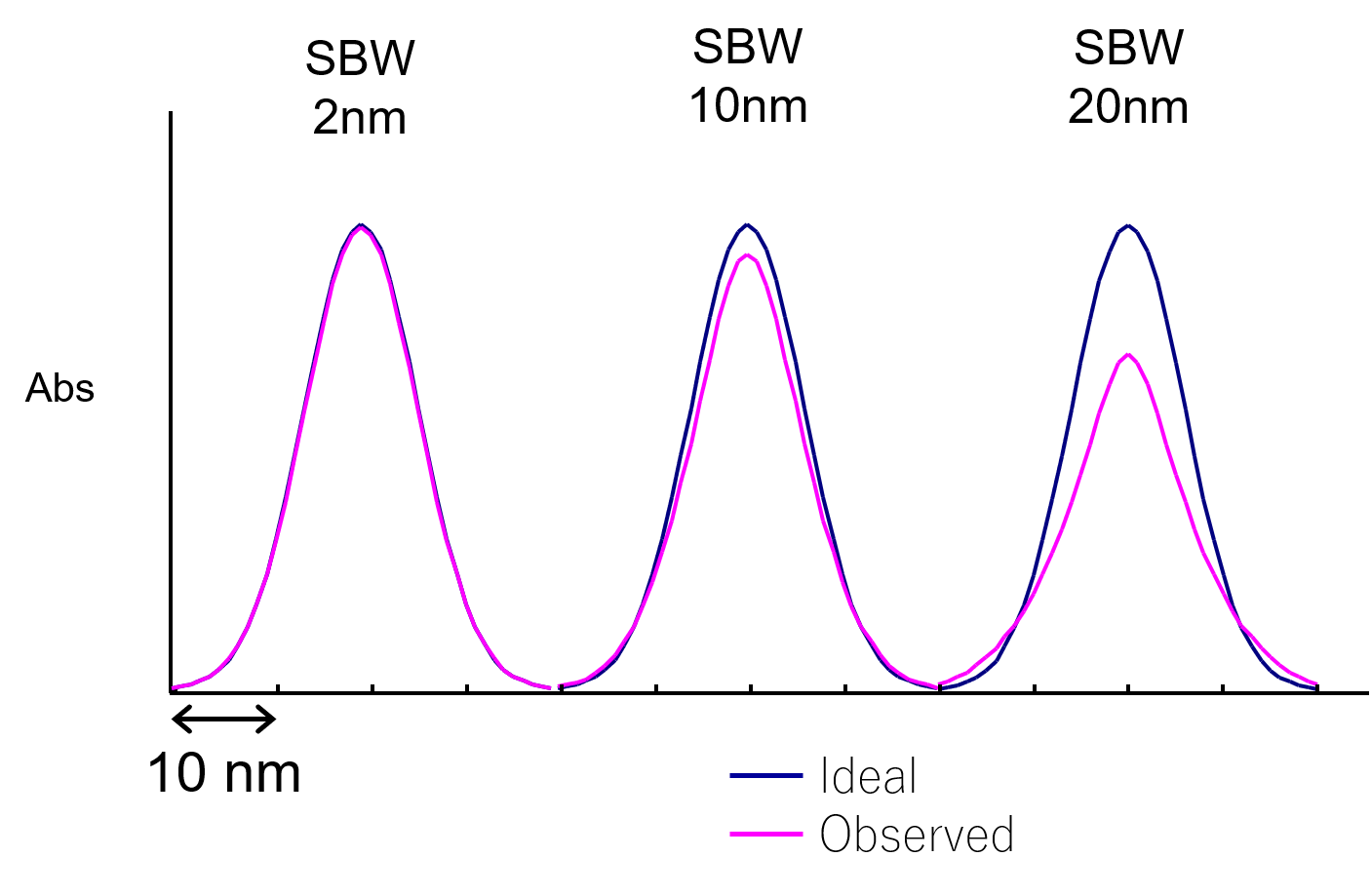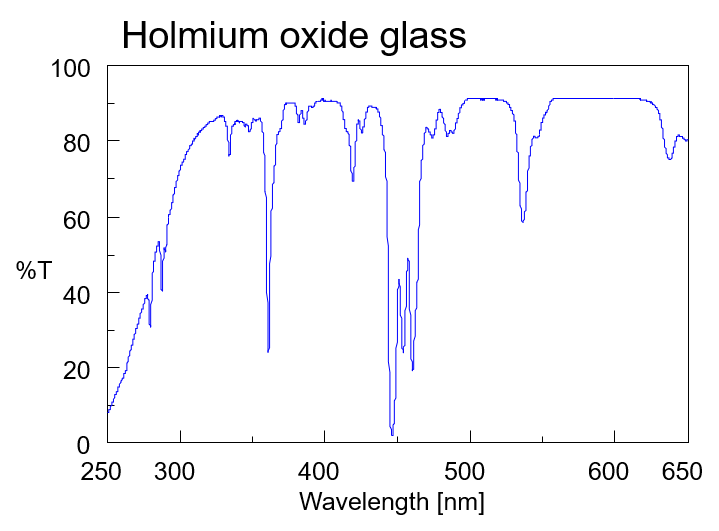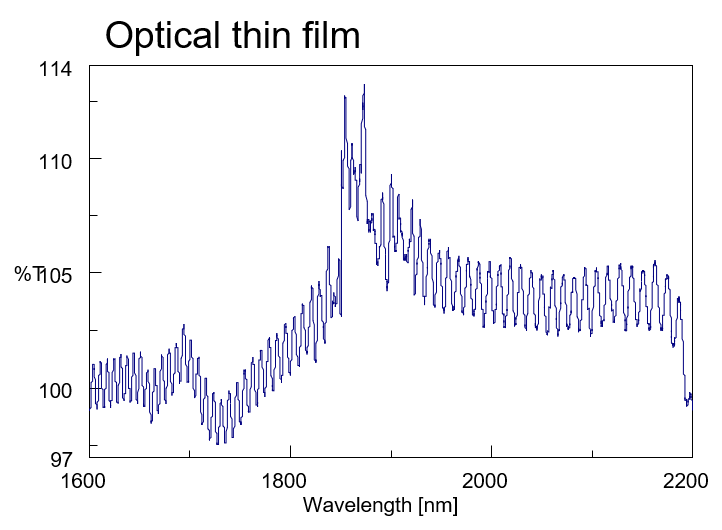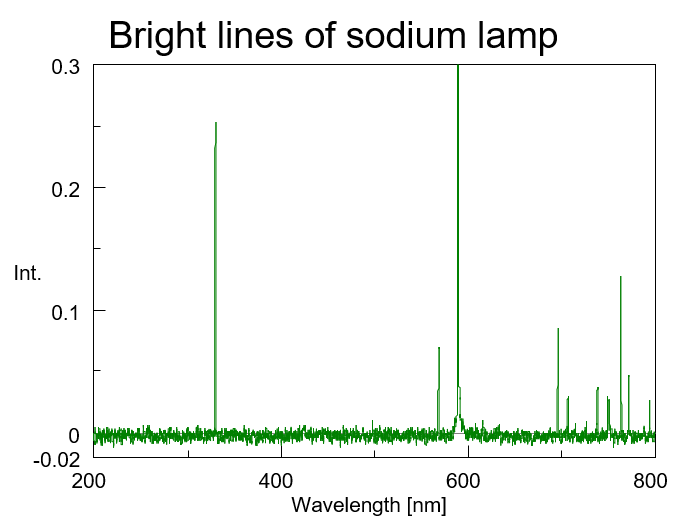# Principles of UV/vis spectroscopy (7) Bandwidth

October 6, 2020

## Bandwidth

The light emerging from a monochromator is not perfectly monochromatic , but contains a narrow range of wavelengths (Fig. 17). When this profile is approximated by a normal distribution, the full width at half maximum is the bandwidth. The smaller the bandwidth, the higher the wavelength purity.Fig. 17 Light extracted from monochromator

## Bandwidth and slit width

The relationship between the bandwidth (Δx) and the slit width (Δλ) is expressed by the following formula:d: groove spacing of diffraction grating
β: diffraction angle
n: diffraction order
f: focal length
Δx: slit width

The bandwidth is set to 1/10 or less of the peak full width at half maximum. Fig. 18 shows the results of measuring absorption peaks with a half-width of 15 nm using bandwidths of 2, 10 and 20 nm. As the bandwidth increases, the peaks collapse and broaden. Generally, when the bandwidth is  1/10 or less of the half width of the sample peak, the measurement error is within 0.5%.Fig. 18 Bandwidth setting and spectrum shape

Decreasing the bandwidth reduces the amount of light and increases the noise. For example, using an integrating sphere to measure an antireflection film, the amount of light detected is small, and is further reduced when the bandwidth is decreased. This is a problem when measuring the absorption edge for an optical filter, the interference waveform for a relatively thick film, a filter for wavelength calibration, and gas absorption and emission (Fig. 19).Fig. 19 Measurement examples where bandwidth is important